Kronig-Penny Model in Higher Dimension

R. B. Laughlin January 24, 2007

(Submitted as coursework for AP272, Stanford University, Winter 2007)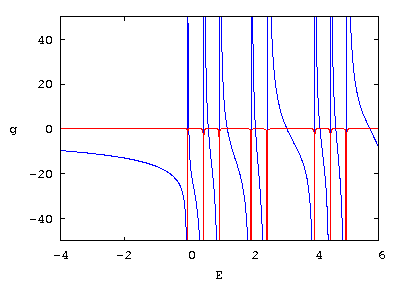Fig. 1: Bare on-site Green's function matrix element g(E + i ε ) for the Kronig-Penny problem on a 2-dimensional square lattice for the case of q = 0. The real and imaginary parts are shown in blue and red. The of the imaginary part locate the free-electron eigenvalues. The eigenvalues of the model occur when 1 = V g(E).

The Kronig-Penny model - electrons moving through a lattice of δ-function potentials - is particularly useful band structure example because it has a simple exact solution. You find it worked out in most solid state textbooks. However, you don't find the higher-dimensional versions worked out as a rule, even though they also have exact solutions, because some extra mathematics is involved, and you don't gain that much more physical insight for your trouble. However, these higher-dimensional models are actually worth studying because they are beautiful examples of eigenvalue problems that are trivial to solve using Green's functions but hopelessly difficult to solve any other way. There are lots of these in physics, many of them extremely interesting.

Let us consider the simplest case of the higher dimensional Kronig-Penny model, the square lattice in two dimensions: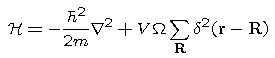Ω is the unit cell volume. V is an energy. The plane wave matrix elements of this hamiltonian are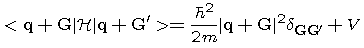If we restrict the number of allowed values of G to a finite number N (with the intention later of taking N to infinity), this hamiltonian becomes a diagonal matrix "perturbed" with a projection operator: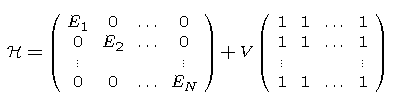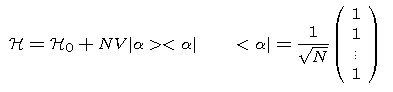All matrices of this form are readily diagonalized by the Koster-Slater "perturbation" technique - a method that is exact and therefore somewhat unfortunately named. The matrix element of the "unperturbed" Greens function connecting the state |α> to itself is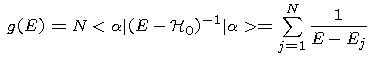It's related exactly to the matrix element of the full Green's function connecting |α> to itself by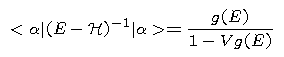Since some matrix elements of the Green's function diverge when E is an eigenvalue, the condition 1 = V g(E) locates some of the eigenvalues. Actually it locates all of them!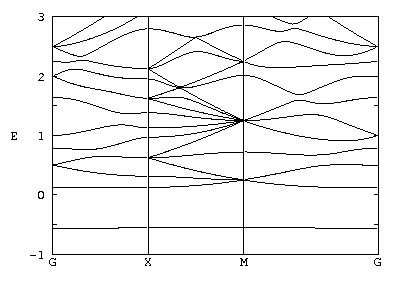Fig. 2: Band structure for the 2-dimensional Kronig Penny model for the case of V = -0.05. Note the very flat band pulled down from the continuum.

Fig. 1 shows what g(E) looks like for the 2-dimensional Kronig-Penny model for q = 0. It has an imaginary part because E+iε has been substituted for E to numerically control the function near its resonances. The fortran source of the code that generated g(E) is available here. The figure was made by gnuplot, the input file of which is available here.

Fig. 2 shows the band structure calculated using this method. It is unremarkable except it complexity - a side effect of the strength of V. The fortran source of the code which generated these bands is available here. The gnuplot input file from which the plot was made is available here.

Now we must address the the problem that limiting N to infinity doesn't make sense. The unperturbed Green's function diverges as ln(N), as can be seen by approximating its sum with an integral: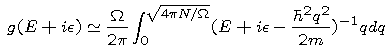The severity of the divergence obviously increases with the number of dimensions. The origin of the difficulty is the δ-function potential, which is unphysically short-ranged in dimension greater than 1. This may be seen by constructing the solution of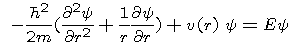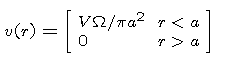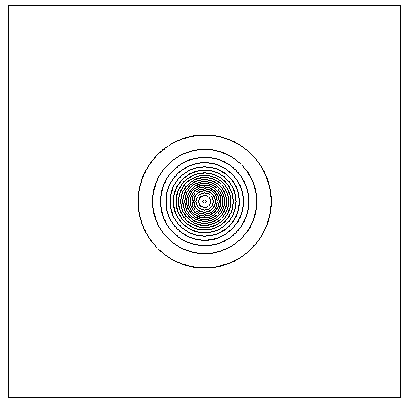Fig. 3: Contour plot of the square of the wavefunction for the lowest band at q = 0. The contours are at integral multiples of 2. The box is one bond length on each side, and locates the unit cell boundary.

and the letting "a" go to zero. Thus, the Kronig-Penny hamiltonian, taken literally, isn't physical. You need to broaden the δ-functions a little. Limiting the calculation to N plane waves accomplishes this broadening.

A particularly interesting feature of this solution is the very flat band pulled down below the continuum. Fig. 3 shows a contour plot of the square of this wavefunction for the case of q = 0. Evidently this wavefunction is a tightly bound s state hugging the δ-function. We have made a tight-binding model! The relative small amplitude of this orbital at the unit cell boundary means that electrons in it can't tunnel very easily to near-neighbor sites. That's why the band is flat. The fortran source of the code that generated the contours is available here. The gnuplot input file from which the plot was made is available here.

The wavefunction is generated by the formula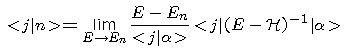This is possible because (1) the eigenstate isn't degenerate and (2) the eigenstate has a nonzero projection on |α>. The off-diagonal Green's function matrix element, in turn, is given byThis result is obtained by appropriately manipulating the inversion equations: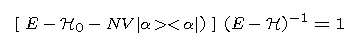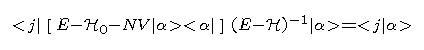Further manipulation yields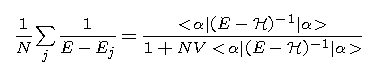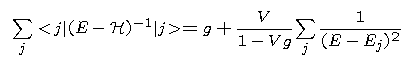The latter demonstrates that this method finds all the energy eigenvalues, not just some of them.

© 2007 R. B. Laughlin. The author grants permission to copy, distribute and display this work in unaltered form, with attribution to the author, for noncommercial purposes only. All other rights, including commercial rights, are reserved to the author.